In this post you will learn such data structure as linked list. This is one of the questions that you can get on the interview quite often.

The first question is what is linked list and how it can be compared to the array. Why is that? Because an array is a sequence of data that we use really often inside Javascript.Linked list has a similar concept.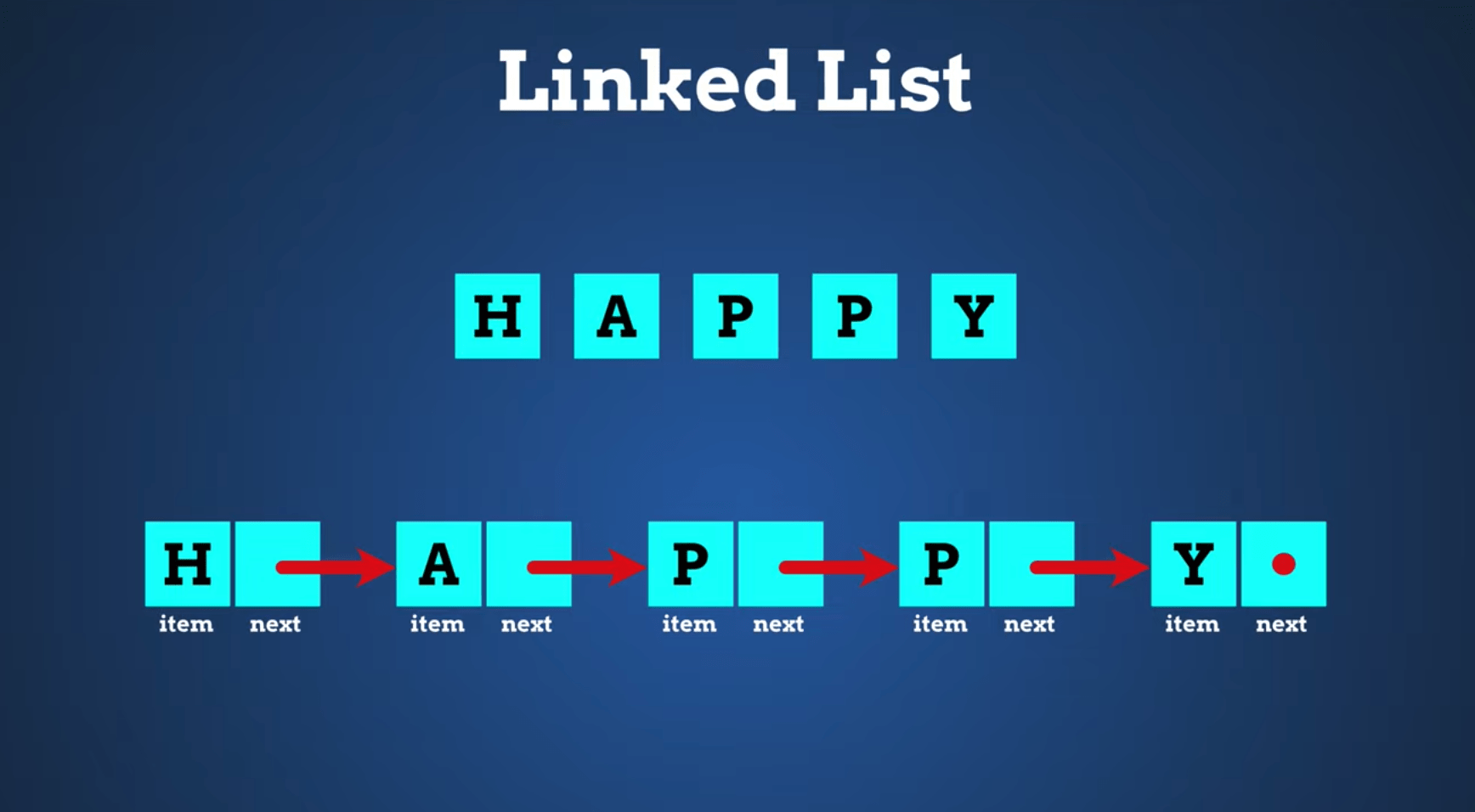Inside linked list we don't store values and indexes like an array but in every single node we store a value and a reference to the next node.

## Performance

So what about performace? Accessing elements in normal array doesn't increase complexity with the amount of elements.In the linked list we can access directly only the first element. All other elements we can get only by accessing the sequence of nodes. Which actually means it increases complexity.
The most important benefit of linked list in comparison to array is inserting and removing elements. It doesn't increase complexity with the amount of elements.
In comparison to linked list in order to remove or add elements to the array we essentially create a new array which is either smaller or bigger. Which means the complexity of it much higher.Getting the size of the array doesn't increase complexity in array. We can achive exactly the same by storing the size of our linked list additionally.

When you should use plain array and when should you use linked list?Almost always you want to use array for most of the cases. Linked list wins only for adding and removing lots of elements.

## Variants to use

It is important to remember that there are 2 different variants to implement linked list. A singly and a doubly.
Singly means that we have just a single direction. Each element has only a reference to the next one.
If we implement additionally functionality to go in the opposite direction by storing reference to previous element then it is called doubly.The goal of this post is to make a singly implementation of linked list.

## Single node

The first thing that we need to implement is an additional class `Node`.
``````class Node {
contructor(value) {
this.value = value;
this.next = null;
}
}
const node = new Node(111);
``````
Here we implemented a `Node` class which stores inside a value and a reference to the next element.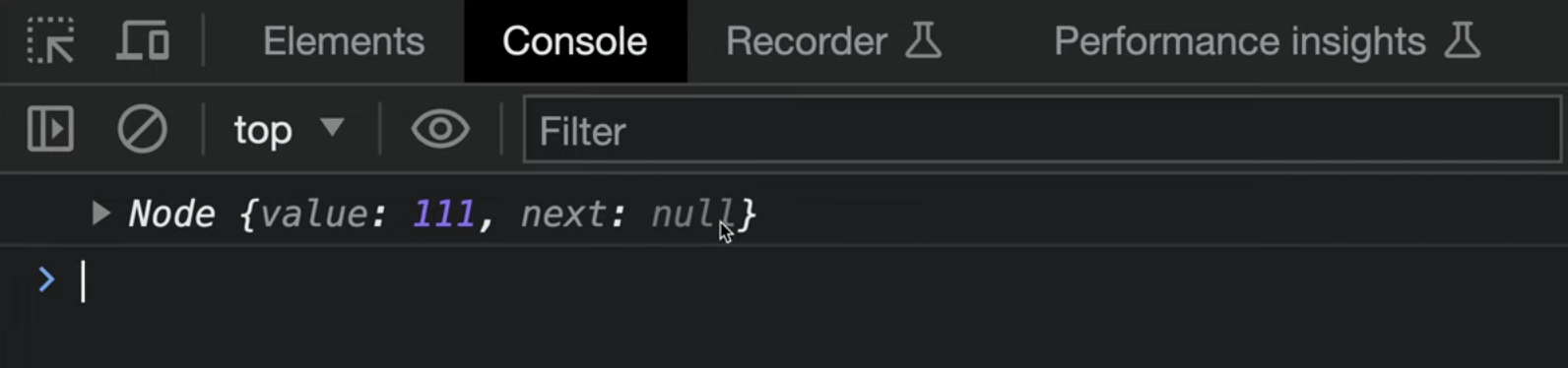As you can see it's just an object with a `value` and `next`.

Now let's implement a basic linked list.
``````class LinkedList {
contructor() {
this.size = 0;
}

const newNode = new Node(value);

if (this.size === 0) {
}

this.size++;
}
}
``````
In our `LinkedList` we store a size property and a head which stores the reference to the next element.In our add element we create a new node and if our linked list is empty we assign the reference of our node to `head`.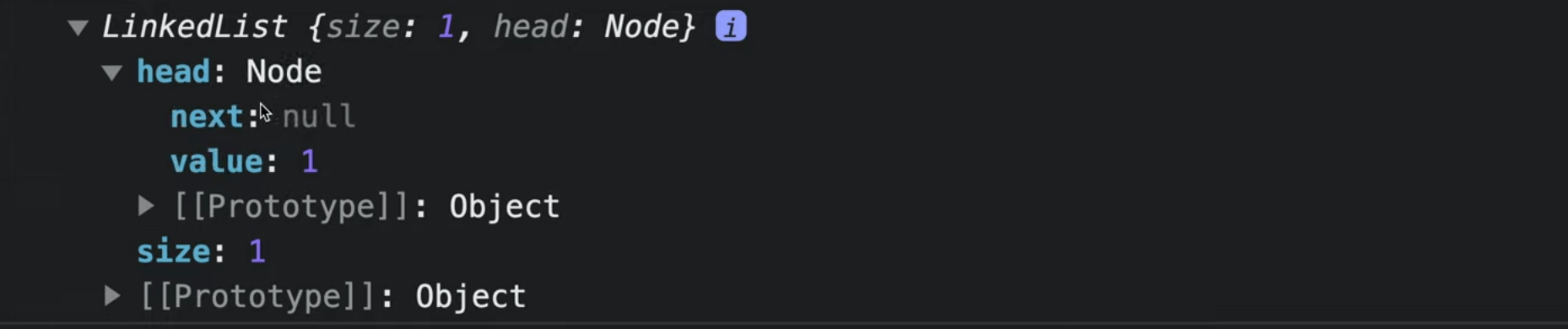As you can see in browser the size is one and in head to store a reference to our node with value 1.So this code saved in the linked list our first node.

## Adding node to not empty list

Now we must add a case when our linked list is not empty.
``````  add (value) {
const newNode = new Node(value);

if (this.size === 0) {
} else {
while (currentNode.next) {
currentNode = currentNode.next;
}
currentNode.next = newNode;
}

this.size++;
}
``````
We need to loop through every single node starting from `this.head`. Every time we assign to `currentNode` the next reference. This is why after our while loop we get have a last node inside `currentNode` and we can save `newNode` inside the `next` property.Let's add one more element to the linked list.
``````const linkedList = new LinkedList();
``````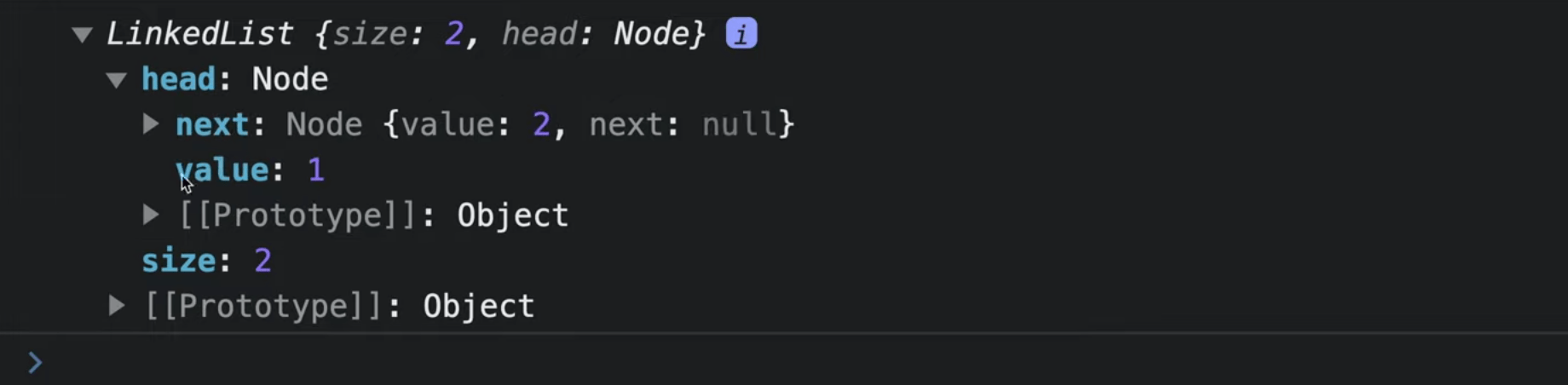As you can see now we have our data as a tree. Inside the first node we have a second node inside `next` property and so on.
The whole linked list is just a nested references from first node to the second, from second to third and so on. The last node doesn't have any reference.

## Getting node by index

Another method that we need is getting a node by index. We can't just get an element by index like in the array. We need to loop through all our elements in order to get an element by index.
``````getByIndex(index) {
let position = 0;

while (position < index) {
currentNode = currentNode.next;
position++;
}

return currentNode;
}
``````
Here we start with `position` zero which is our first element. We loop with `while` und the previous element to our index. Every time we assign a node inside our `currentNode` property.
``````console.log(linkedList.getByIndex(1))
``````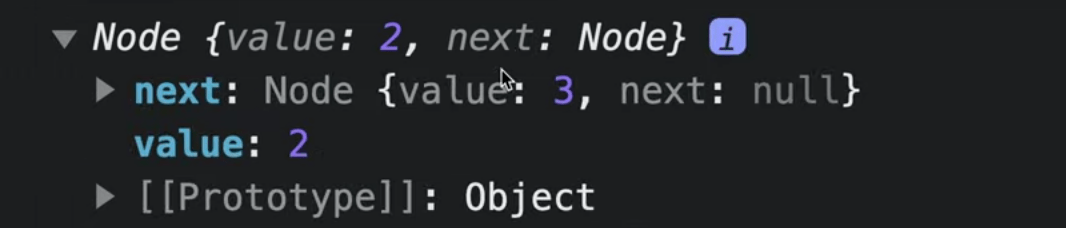As you can see in browser we successfully got our element.

## Inserting a node

Now we must implement add by index function. This is one of the most important functions because this is exactly why linked list shines. It allows us to update our linked list without increasing complexity.
``````addByIndex(index, value) {
const newNode = new Node(value);

if (this.size === 0) {
return;
}

const previousNode = this.getByIndex(index - 1);
newNode.next = previousNode.next;
previousNode.next = newNode;
this.size++;
}
``````
Here we have 2 cases. Our linked list is either empty or not. If it is empty we simply assign a new node to `head`. If it is not empty we use `getByIndex` property to get the previous element. Then we update the references to the next nodes so we squeeze our new node between them.
``````linkedList.addByIndex(1, 5)
``````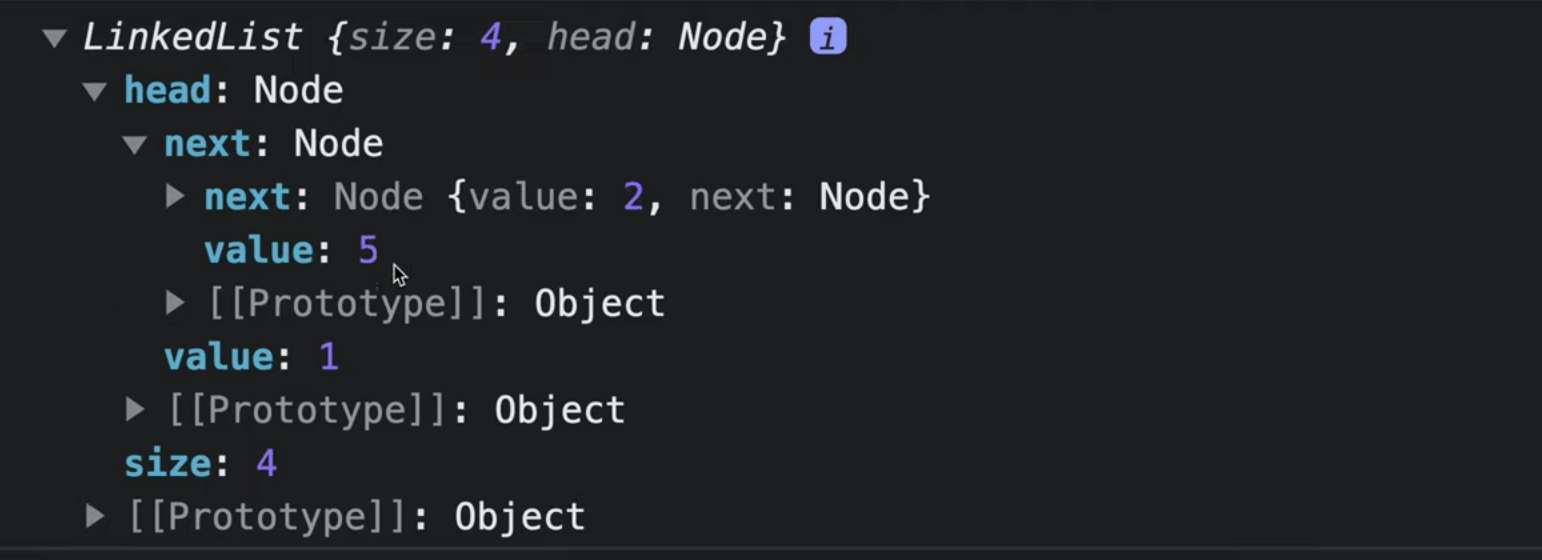Here is our linked list and we can see that now after value `1` we have value `5` and only then value `2`.

## Remove node

The last method that we want to implement is removing node by index. It will work in exactly the same way like adding a node.
``````removeByIndex(index) {